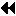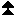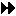## 9.2.7. Querying File ParametersUp: File Manipulation Next: File Info Previous: Querying the Size of a File

MPI_FILE_GET_GROUP(fh, group)
[ IN fh] file handle (handle)
[ OUT group] group which opened the file (handle)

int MPI_File_get_group(MPI_File fh, MPI_Group *group)
MPI_FILE_GET_GROUP(FH, GROUP, IERROR)
INTEGER FH, GROUP, IERROR
MPI::Group MPI::File::Get_group() const

MPI_FILE_GET_GROUP returns a duplicate of the group of the communicator used to open the file associated with fh. The group is returned in group. The user is responsible for freeing group.

MPI_FILE_GET_AMODE(fh, amode)
[ IN fh] file handle (handle)
[ OUT amode] file access mode used to open the file (integer)

int MPI_File_get_amode(MPI_File fh, int *amode)
MPI_FILE_GET_AMODE(FH, AMODE, IERROR)
INTEGER FH, AMODE, IERROR
int MPI::File::Get_amode() const

MPI_FILE_GET_AMODE returns, in amode, the access mode of the file associated with fh.

Example In Fortran 77, decoding an amode bit vector will require a routine such as the following:

```SUBROUTINE BIT_QUERY(TEST_BIT, MAX_BIT, AMODE, BIT_FOUND)
!
!   TEST IF THE INPUT TEST_BIT IS SET IN THE INPUT AMODE
!   IF SET, RETURN 1 IN BIT_FOUND, 0 OTHERWISE
!
INTEGER TEST_BIT, AMODE, BIT_FOUND, CP_AMODE, HIFOUND
BIT_FOUND = 0
CP_AMODE = AMODE
100  CONTINUE
LBIT = 0
HIFOUND = 0
DO 20 L = MAX_BIT, 0, -1
MATCHER = 2**L
IF (CP_AMODE .GE. MATCHER .AND. HIFOUND .EQ. 0) THEN
HIFOUND = 1
LBIT = MATCHER
CP_AMODE = CP_AMODE - MATCHER
END IF
20  CONTINUE
IF (HIFOUND .EQ. 1 .AND. LBIT .EQ. TEST_BIT) BIT_FOUND = 1
IF (BIT_FOUND .EQ. 0 .AND. HIFOUND .EQ. 1 .AND. &
CP_AMODE .GT. 0) GO TO 100
END
```

This routine could be called successively to decode amode, one bit at a time. For example, the following code fragment would check for MPI_MODE_RDONLY.

```CALL BIT_QUERY(MPI_MODE_RDONLY, 30, AMODE, BIT_FOUND)
IF (BIT_FOUND .EQ. 1) THEN
PRINT *, ' FOUND READ-ONLY BIT IN AMODE=', AMODE
ELSE